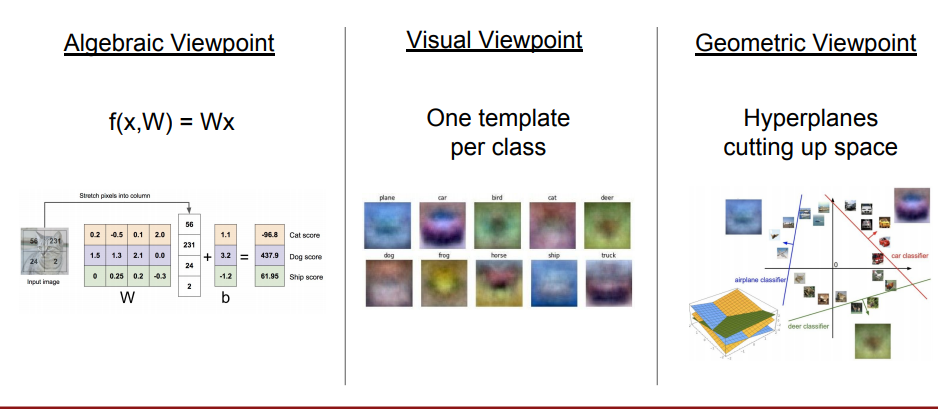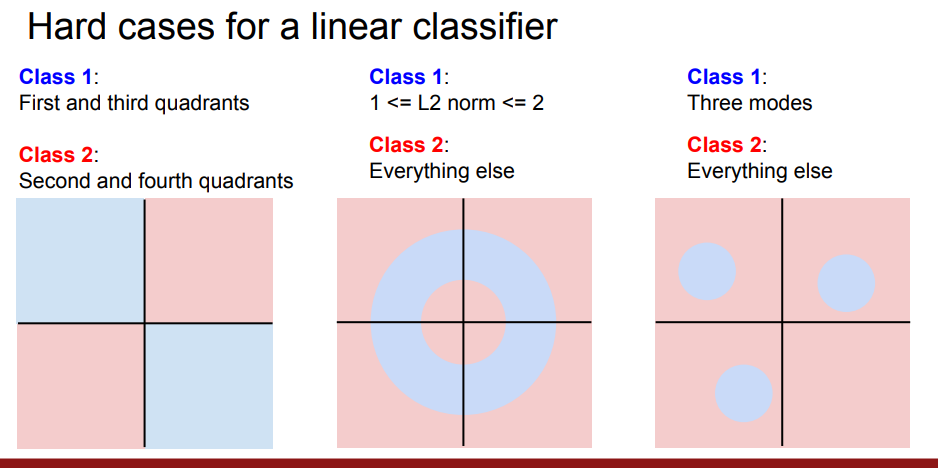# 图像分类

ppt

## 1 数据驱动方法

1. 一些像素的简单偏移或者变化，会使整张图片有改变；
2. 物体所在的环境，如光线，位置会有变化；
3. 物体本身有不同的姿态；
4. 可能有障碍物遮挡物体；

• 该方法的train步骤只是单纯地记录下所有的图片和标签。
• 该方法的predict步骤对每个新的图像，找出train中和它最相似的图像，将它的图像标签赋给新图像。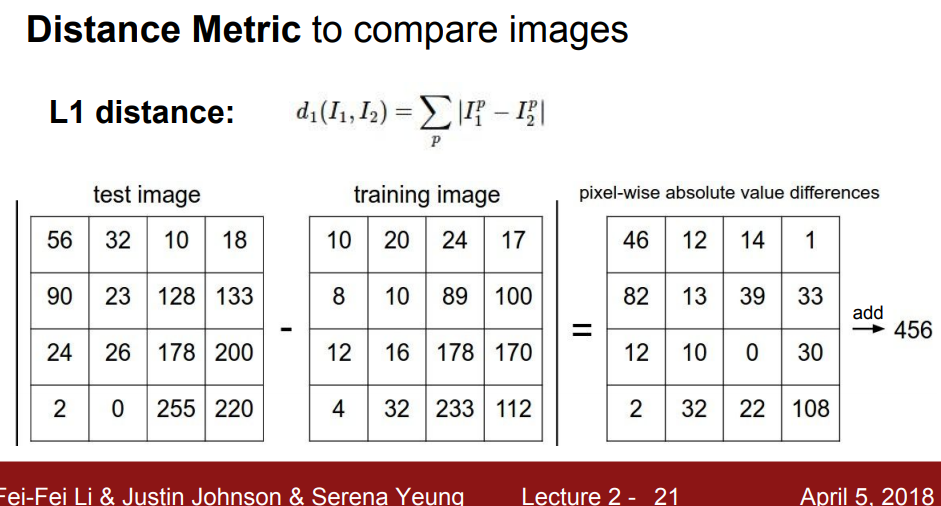class NearestNeighbor:
def __init__(self):
pass

def train(self,X,y):
'''X is N*D where each row in a dimension, y is a one-dimension '''
#the nearesr neighrbor algorithm simply remember all training data
self.X_tr = X
self.y_tr = y

def test(self,X):
'''X is M*D where each row is an example we wish to predict label for'''
num_test = X.shape
Ypred = np.zeros(num_test,dtype = self.y_tr.dtype)
for i in range(num_test):
distances = np.sum(np.abs(self.X_tr-X[i,:]),xis=1)
min_index = np.argmin(distances)
Ypred[i] = self.y_tr[min_index]
return Ypred



Nearest Neighbor训练时的时间复杂度为$O(1)$，预测的时间复杂度为$O(n)$，这是不好的。因为我们构件模型的目的在于希望它能快速地预测，反而训练的时间就算长一些也可以接受。

## 2 KNN算法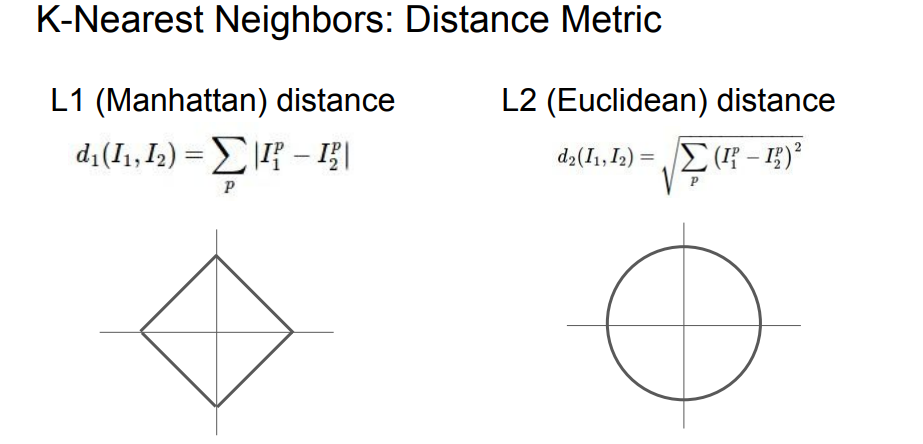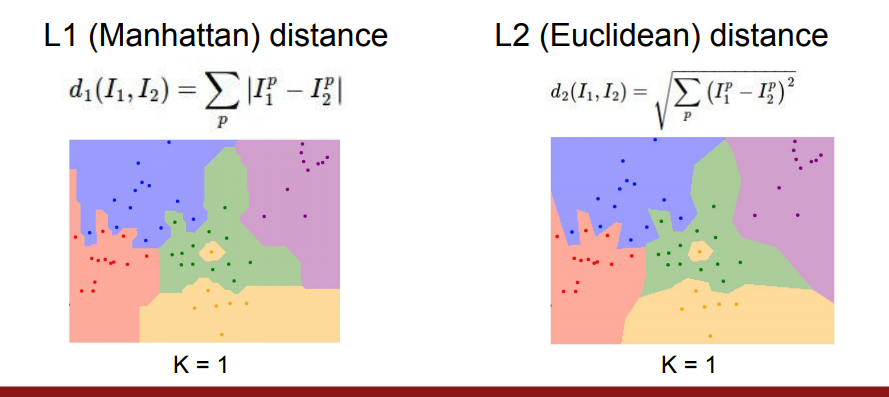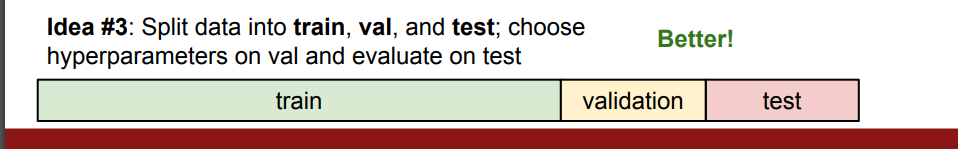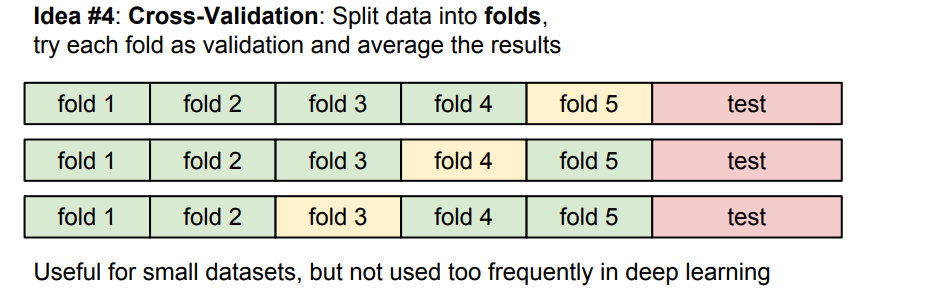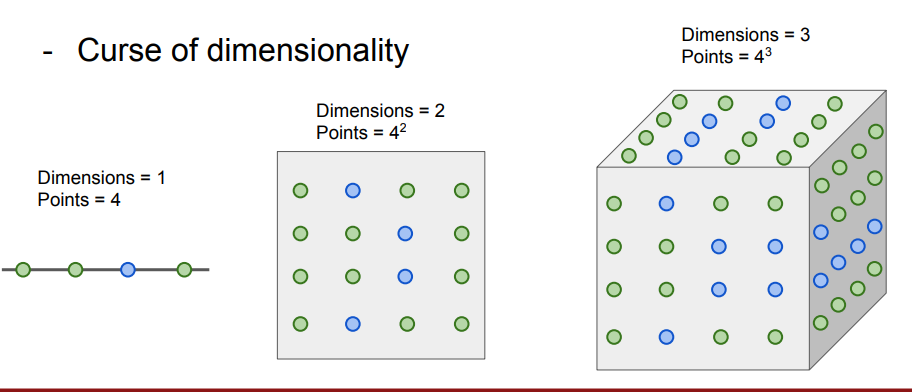## 3 线性分类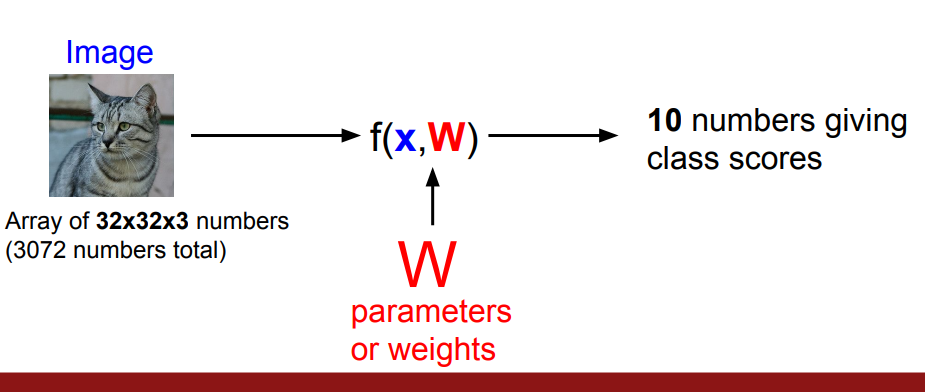$x$$W$相乘，是一种直观和简单的方法，线性分类器也是这么做的。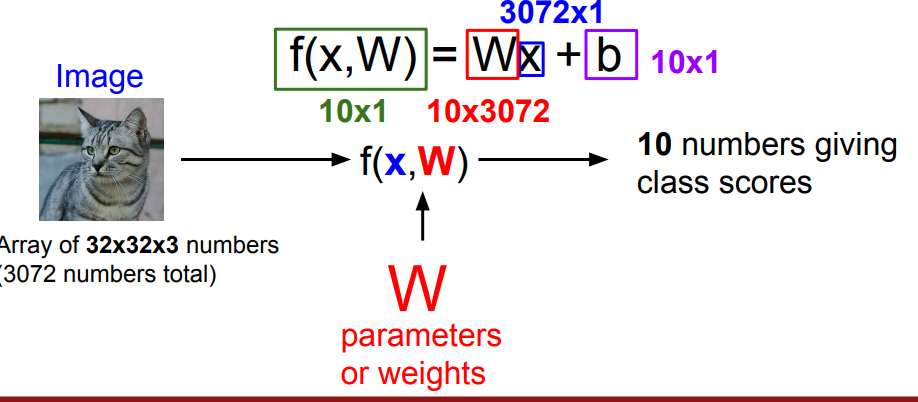$32 \times 32 \times 3$的图片拉伸成$3072 \times 1$的向量$x$，输出$f(w,X)$$10 \times 1$的向量，所以$W$的形状就是一个$10 \times 3072$的矩阵。这里我们可以加一个偏执常数向量$b$，表示给每一个类加偏置，比如训练数据中猫的图片远远多于狗的图片，那么猫的偏置值会大一些。